# Ellipse

An ellipse is the locus of all those points in a plane such that the sum of their distances from two fixed points in the plane, is constant. The fixed points are known as the foci (singular focus), which are surrounded by the curve. The fixed line is directrix and the constant ratio is eccentricity of ellipse. Eccentricity is a factor of the ellipse, which demonstrates the elongation of it and is denoted by ‘e’.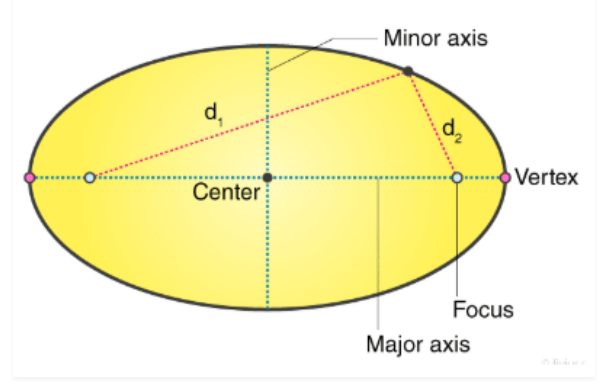The shape of the ellipse is in an oval shape and the area of an ellipse is defined by its major axis and minor axis. Area of ellipse = πab, where a and b are the length of semi-major and semi-minor axis of an ellipse. Ellipse is similar to other parts of the conic section such as parabola and hyperbola, which are open in shape and unbounded.

## Definition

An ellipse if we speak in terms of locus, it is the set of all points on an XY-plane, whose distance from two fixed points (known as foci) adds up to a constant value.

The ellipse is one of the conic sections, that is produced, when a plane cuts the cone at an angle with the base. If the cone is intersected by the plane, parallel to the base, then it forms a circle.

### Ellipse Shape

In geometry, an ellipse is a two-dimensional shape, that is defined along its axes. An ellipse is formed when a cone is intersected by a plane at an angle with respect to its base.

It has two focal points. The sum of the two distances to the focal point, for all the points in curve, is always constant.

A circle is also an ellipse, where the foci are at the same point, which is the center of the circle.

### Major and Minor Axis

Ellipse is defined by its two-axis along x and y-axis:

• Major axis
• Minor Axis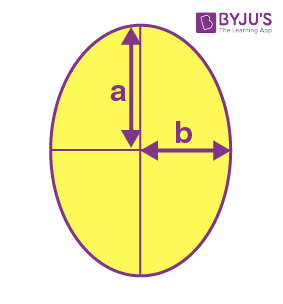The major axis is the longest diameter of the ellipse (usually denoted by ‘a’), going through the center from one end to the other, at the broad part of the ellipse. Whereas the minor axis is the shortest diameter of ellipse (denoted by ‘b’), crossing through the center at the narrowest part.

Half of major axis is called semi-major axis and half of minor axis is called semi-minor axis.

## Properties

• Ellipse has two focal points, also called foci.
• The fixed distance is called a directrix.
• The eccentricity of ellipse lies between 0 to 1. 0≤e<1
• The total sum of each distance from the locus of an ellipse to the two focal points is constant
• Ellipse has one major axis and one minor axis and a center

## Eccentricity of the Ellipse

The ratio of distances from the center of the ellipse from either focus to the semi-major axis of the ellipse is defined as the eccentricity of the ellipse.

The eccentricity of ellipse, e = c/a

Where c is the focal length and a is length of the semi-major axis.

Since c ≤ a the eccentricity is always greater than 1 in the case of an ellipse.
Also,
c2 = a2 – b2
Therefore, eccentricity becomes:
e = √(a2 – b2)/a
e = √[(a2 – b2)/a2] e = √[1-(b2/a2)]

## Video Lesson

### Divisibility Models## Ellipse Equation

When the centre of the ellipse is at the origin (0,0) and the foci are on the x-axis and y-axis, then we can easily derive the ellipse equation.

The equation of the ellipse is given by;

x2/a2 + y2/b2 = 1

### Derivation of Ellipse Equation

Now, let us see how it is derived.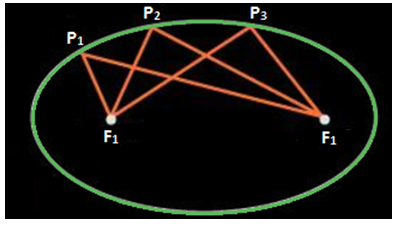.

The above figure represents an ellipse such that P1F1 + P1F2 = P2F1 + P2F2 = P3F1 + P3F2 is a constant. This constant is always greater than the distance between the two foci. When both the foci are joined with the help of a line segment then the mid-point of this line segment joining the foci is known as the center, O represents the center of the ellipse in the figure given below: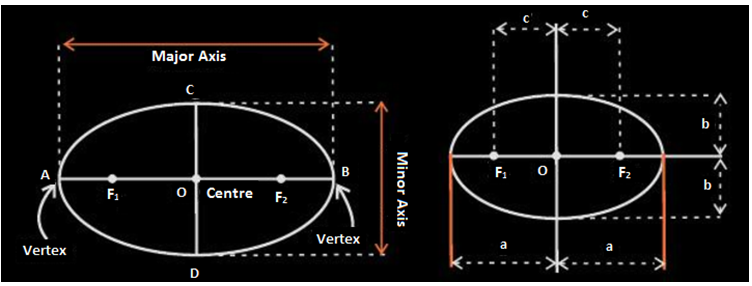The line segment passing through the foci of the ellipse is the major axis and the line segment perpendicular to the major axis and passing through the center of the ellipse is the minor axis. The end points A and B as shown are known as the vertices which represent the intersection of major axes with the ellipse. ‘2a’ denotes the length of the major axis and ‘a’ is the length of the semi-major axis. ‘2b’ is the length of the minor axis and ‘b’ is the length of the semi-minor axis. ‘2c’ represents the distance between two foci.

### Proof:

Let us consider the end points A and B on the major axis and points C and D at the end of the minor axis.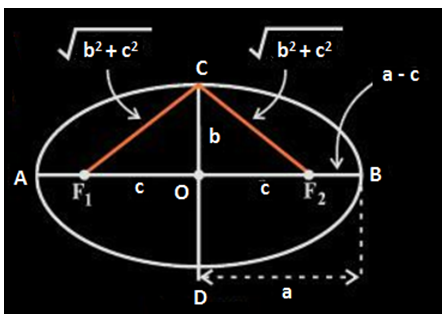The sum of distances of B from F1 is F1B + F2B = F1O + OB + F2B (From the above figure)

⇒ c + a + a – c = 2a

The sum of distances from point C to F1 is F1C + F2C

⇒ F1C + F2C = √(b2 + c2) + √(b2 + c2) = 2√(b2 + c2)

By definition of ellipse;

2√(b2 + c2) = 2a

a = √(b2 + c2)

⇒ a2 = b2 + c2

c2 = a2 – b2

Special Cases:

• If c = 0 then F1 and F2, i.e. both foci merge together with center of ellipse. Also a2 becomes equal to b2, i.e. a = b so now we get a circle in this case.
• If c = a then b becomes 0 and we get a line segment F1F2.

## Standard Equation of Ellipse

The simplest method to determine the equation of an ellipse is to assume that centre of the ellipse is at the origin (0, 0) and the foci lie either on x- axis or y-axis of the Cartesian plane as shown below: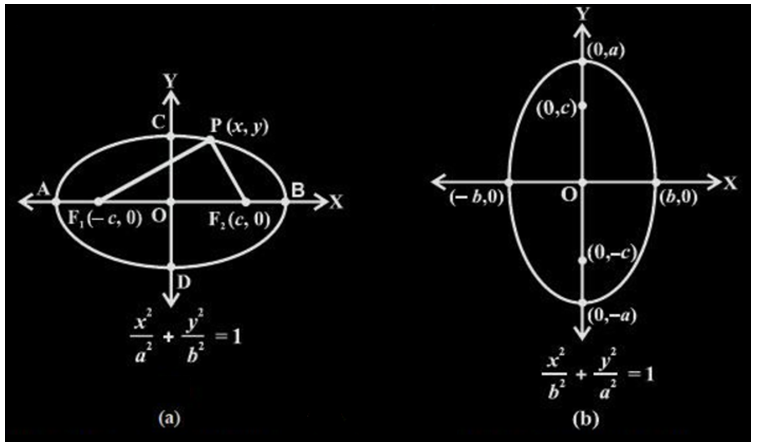Both the foci lie on the x- axis and center O lies at the origin.

Let us consider the figure (a) to derive the equation of an ellipse. Let the coordinates of F1 and F2 be (-c, 0) and (c, 0) respectively as shown. Let us consider a point P(x, y) lying on the ellipse such that P satisfies the definition i.e. the sum of distances of P from F1 and F2 in the plane is a constant 2a.

⇒ PF1 + PF2 = 2a – – – (1)

Using distance formula the distance can be written as: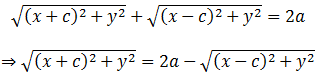Squaring and simplifying both sides we get;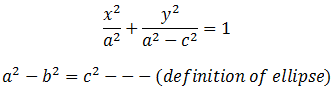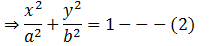Now since P lies on the ellipse it should satisfy equation 2 such that 0 < c < a.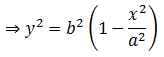Thus,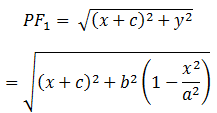On simplifying,

PF1 = a + (c/a)x

Similarly,

PF= a – (c/a)x

Therefore,

PF+ PF= 2a

Therefore the equation of the ellipse with centre at origin and major axis along the x-axis is: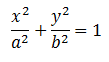where –a ≤ x ≤ a.

Similarly, the equation of the ellipse with center at origin and major axis along the y-axis is: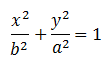where –b  ≤ y ≤ b.

## PDF for Formulas

Click here to download the equations and formulas of an ellipse – Download PDF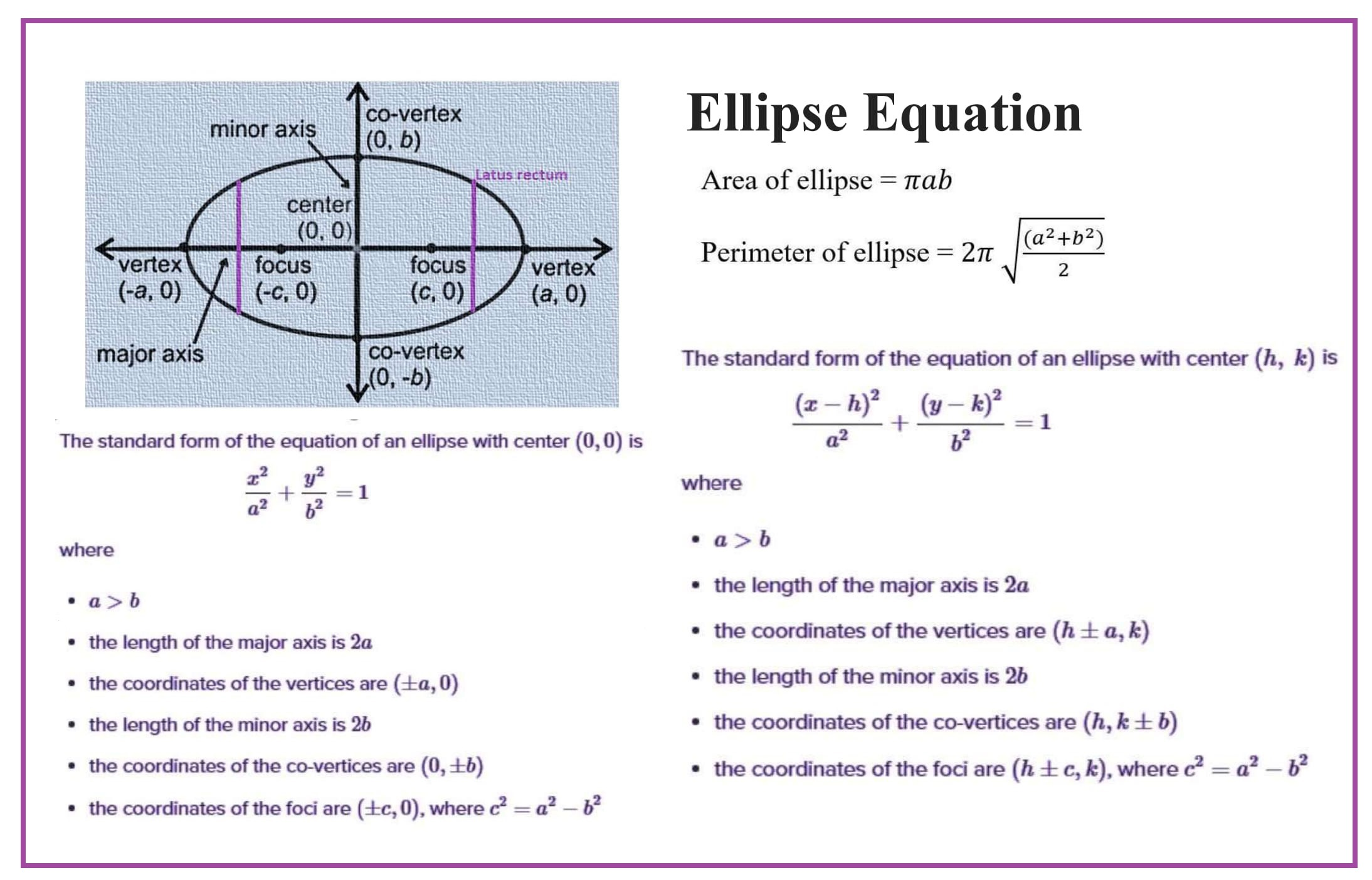## Ellipse Formula

As we know, an ellipse is a closed-shape structure in a two-dimensional plane. Hence, it covers a region in a 2D plane. So, this bounded region of the ellipse is its area.  The shape of the ellipse is different from the circle, hence the formula for its area will also be different.

### Area of Ellipse

Area of the circle is calculated based on its radius, but the area of the ellipse depends on the length of the minor axis and major axis. Check more here: Area of an ellipse.

Area of the circle = πr2

And,

Area of the ellipse = π x Semi-Major Axis x Semi-Minor Axis

 Area of the ellipse = π.a.b

### Perimeter of Ellipse

The perimeter of an ellipse is the total distance run by its outer boundary. For a circle, it is easy to find its circumference, since the distance from the center to any point of locus of circle is same. This distance is called radius.

But in the case of an ellipse, we have two axis, major and minor, that crosses through the center and intersects. Hence, we use an approximation formula to find the perimeter of an ellipse, given by:

$$\begin{array}{l}p \approx 2 \pi \sqrt{\frac{a^{2}+b^{2}}{2}}\end{array}$$

Where a and b are the length of semi-major and semi-minor axes respectively.

### Latus Rectum

The line segments perpendicular to the major axis through any of the foci such that their endpoints lie on the ellipse are defined as the latus rectum.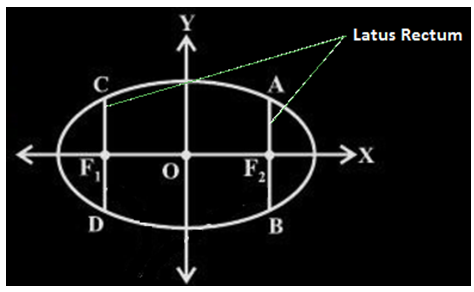The length of the latus rectum is 2b2/a.

L = 2b2/a

where a and b are the length of the minor axis and major axis.

### Problem and Solution

Q.1: If the length of the semi major axis is 7cm and the semi minor axis is 5cm of an ellipse. Find its area.

Solution: Given, length of the semi-major axis of an ellipse, a = 7cm

length of the semi-minor axis of an ellipse, b = 5cm

By the formula of area of an ellipse, we know;

Area = π x a x b

Area = π x 7 x 5

Area = 35 π

or

Area = 35 x 22/7

Area = 110 cm2

## Frequently Asked Questions – FAQs

### What is an ellipse?

An ellipse is a curve formed by a plane, that intersects a cone at an angle with respect to the base. It is a curve surrounded by two focal points. Ellipse is a locus of points, the sum of whose distances from two foci is constant.

### What is major and minor axis of ellipse?

The major axis of ellipse lies along x-axis and is the longest width across it. It has length equal to 2a. The endpoints are the vertices of major axis, having coordinates (h±a,k).
Minor axis lies along y-axis and is smallest width across it. It has length equal to 2b. The endpoints are the vertices of minor axis having coordinates (h, k±b).

### What is directrix and eccentricity of ellipse?

In ellipse, the fixed line parallel to minor axis, at a distance of d from the center is called directrix of ellipse. Eccentricity (e) is measured as the elongation of ellipse. The value of ‘e’ lies between 0 and 1, for ellipse.

### What is the area of ellipse?

The area of ellipse is the region covered by the shape in two-dimensional plane. It is given by:
Area = πab
Where a and b is semi-major axis and semi-minor axis respectively.

### What is the equation of ellipse?

The equation of ellipse is given by:
(x2/a2)+(y2/b2) = 1